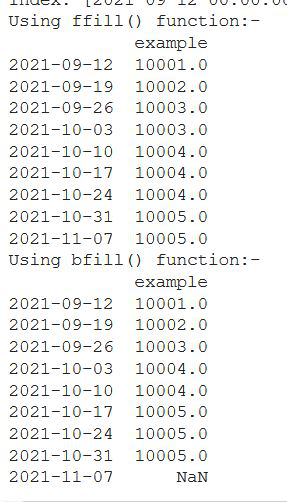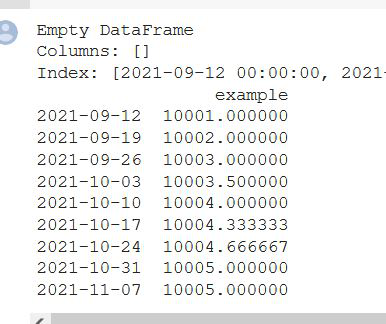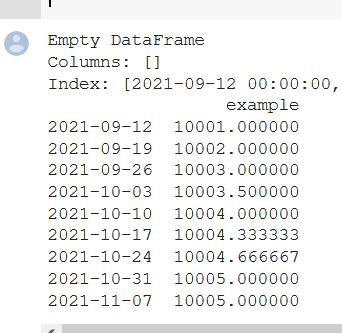Open in App
Not now

# How to deal with missing values in a Timeseries in Python?

• Difficulty Level : Medium
• Last Updated : 05 Nov, 2021

In this article, we will discuss how to deal with missing values in a time series using the Python programming language.

Time series is a sequence of observations recorded at regular time intervals. Time series analysis can be useful to see how a given asset, security, or economic variable changes over time. Another big question here is why we need to deal with missing values in the dataset and why the missing values are present in the data?

• The handling of missing data is very important during the preprocessing of the dataset as many machine learning algorithms do not support missing values.
• Time series are subject to have missing points due to problems in reading or recording the data.

Why can’t we change the missing values with global mean because the time series data might have some like seasonality or trend?  A conventional method such as mean and mode imputation, deletion, and other methods are not good enough to handle missing values as those methods can cause bias to the data. Estimation or imputation of the missing data with the values produced by some procedures or algorithms can be the best possible solution to minimize the bias effect of the conventional method of the data. So that at last, the data will be completed and ready to use for another step of analysis or data mining.

## Method 1: Using ffill() and bfill() Method

The method fills missing values according to sequence and conditions. It means that the method replaces ‘nan’s value with the last observed non-nan value or the next observed non-nan value.

1. backfill – bfill : according to the last observed value
2. forwardfill – ffill : according to the next observed value

## Python3

 `# import the libraries``import` `pandas as pd``import` `numpy as np`` ` `# dataframe with index as timeseries``time_sdata ``=` `pd.date_range(``"09/10/2021"``, periods``=``9``, freq``=``"W"``)`` ` `df ``=` `pd.DataFrame(index``=``time_sdata)``print``(df)`` ` `# there are four missing values``df[``"example"``] ``=` `[``10001.0``, ``10002.0``, ``10003.0``, np.nan,``                 ``10004.0``, np.nan, np.nan, ``10005.0``, np.nan]`` ` `gfg1 ``=` `df.ffill()``print``(``"Using ffill() function:-"``)``print``(gfg1)`` ` `# here we are doing Backfill Missing Values``# in the output the last value has NaN because ``# there is no backward value for that``gfg2 ``=` `df.bfill()``print``(``"Using bfill() function:-"``)``print``(gfg2)`

Output:## Method 2: Using Interpolate() Method

The method is more complex than the above fillna() method. It consists of different methodologies, including ‘linear’, ‘quadratic’, ‘nearest’. Interpolation is a powerful method to fill missing values in time-series data. Go through the below link provided for a few more examples.

## Python3

 `# import the libraries``import` `pandas as pd``import` `numpy as np`` ` `# dataframe with index as timeseries``time_sdata ``=` `pd.date_range(``"09/10/2021"``, periods``=``9``, freq``=``"W"``)`` ` `df ``=` `pd.DataFrame(index``=``time_sdata)``print``(df)`` ` `# there are four missing values``df[``"example"``] ``=` `[``10001.0``, ``10002.0``, ``10003.0``, np.nan,``                 ``10004.0``, np.nan, np.nan, ``10005.0``, np.nan]`` ` `# using interpolate() to fill the missing ``# values in a specific order``# dealing with missing values``dataframe1 ``=` `df.interpolate()``print``(dataframe1)`

Output:## Method 3: Using Interpolate() Method with limit parameter

This is the maximum number of consecutive NaN values to forward/backward fill. In other words, if there is a gap with more than this number of consecutive NaNs, it will only be partially filled.

Syntax:

DataFrame.interpolate(method=’linear’, axis=0, limit=None, inplace=False, limit_direction=None, limit_area=None, downcast=None, **kwargs)

Note: Only method=’linear’ is supported for DataFrame/Series with a MultiIndex.

## Python3

 `# import the libraries``import` `pandas as pd``import` `numpy as np`` ` `# dataframe with index as timeseries``time_sdata ``=` `pd.date_range(``"09/10/2021"``, periods``=``9``, freq``=``"W"``)`` ` `df ``=` `pd.DataFrame(index``=``time_sdata)``print``(df)`` ` `# there are four missing values``df[``"example"``] ``=` `[``10001.0``, ``10002.0``, ``10003.0``, np.nan,``                 ``10004.0``, np.nan, np.nan, ``10005.0``, np.nan]`` ` `# Interpolating Missing Values to two values``dataframe ``=` `df.interpolate(limit``=``2``, limit_direction``=``"forward"``)``print``(dataframe)`

Output:My Personal Notes arrow_drop_up